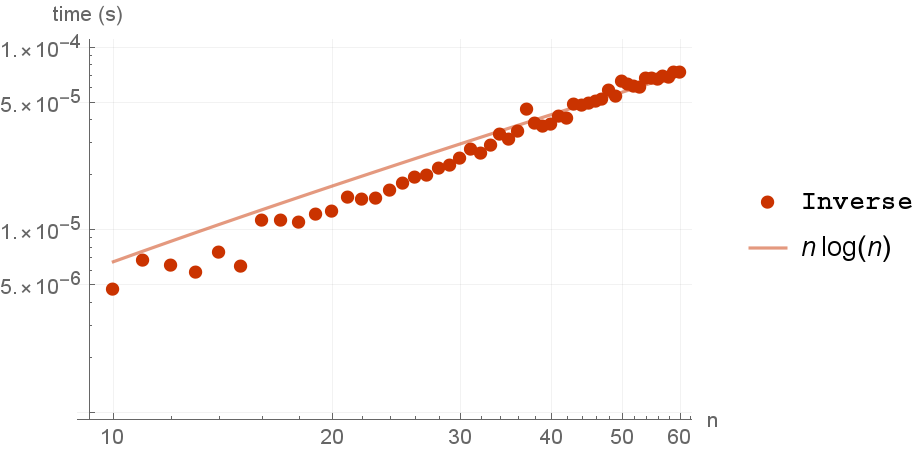# BenchmarkPlot

Contributed by: Taliesin Beynon

Plot the timings of a benchmark

 ResourceFunction["BenchmarkPlot"][f,g] plots the time taken to execute f with input g[n] against integer n, where the n are chosen automatically. ResourceFunction["BenchmarkPlot"][f,g, {n1,n2,…}] uses the explicit ni to generate input g for f. ResourceFunction["BenchmarkPlot"][{f1,f2, …},…] plots the time taken for each of the fi. ResourceFunction["BenchmarkPlot"][data, …] plots the results of a previous ResourceFunction["EvaluateBenchmark"] computation.

## Details and Options

ResourceFunction["BenchmarkPlot"] effectively uses Monitor.

## Examples

### Basic Examples

Get a plot for the inverse of random matrices:

 In:=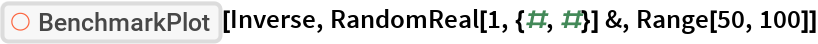Out=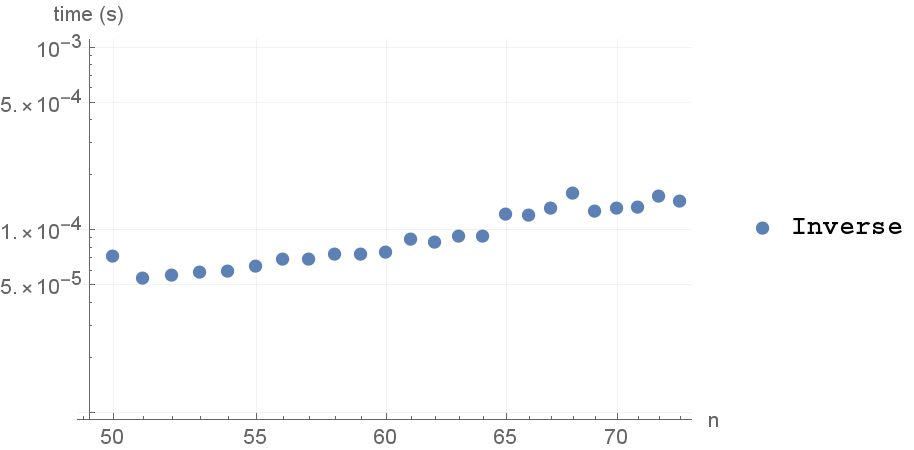Get a plot for the data of a previous benchmark:

 In:=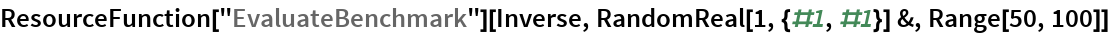Out=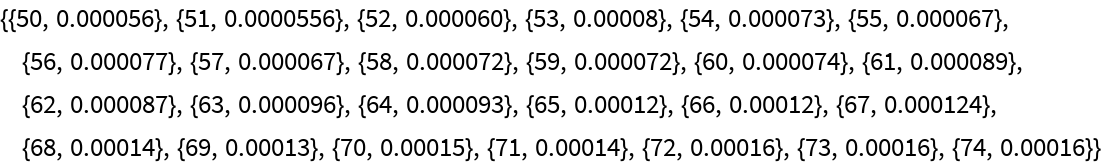In:=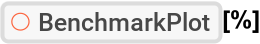Out=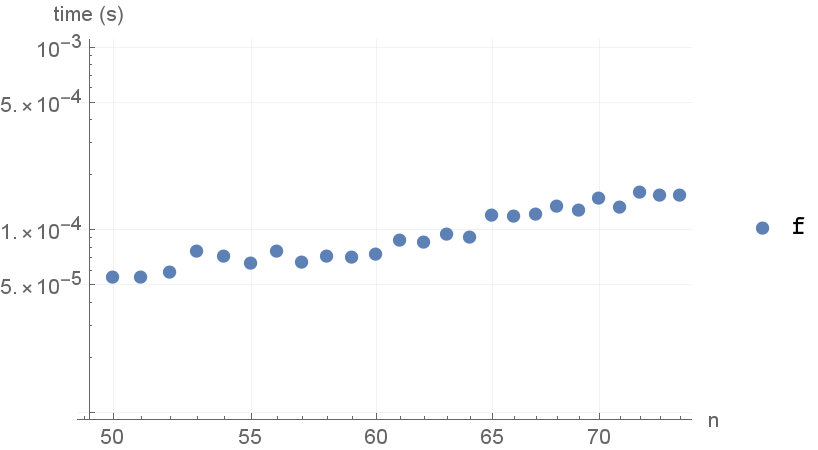### Options

Get a fit for the data:

 In:=Out=